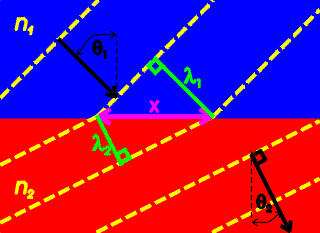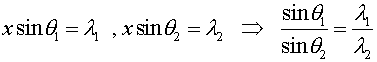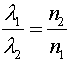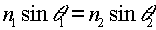Snell's law

Light rays that cross a surface from one medium into another that has a different index of refraction will change speeds when they cross the surface, from c/n1 to c/n2 as discussed previously. As a result, they also change direction by an amount that depends on the indices of refraction. The formula that relates the angles is known as Snell's Law.Snell's law can easily be derived using the sketch on the right which shows light incident from the blue medium at an angle of incidence, q1, that enters the red medium with an angle of refraction, q2. The yellow lines represent the wave fronts. Some geometry shows:From the previous page one knows that the wavelengths are inversely proportional to the indices of refraction:Combining the last two equations gives Snell's law:The bending of light rays according to Snell's law is the basic principle that underlies the operation of optical systems such as the lenses used for eyeglasses, telescopes and microscopes.

Refraction index      examples        Lecture index